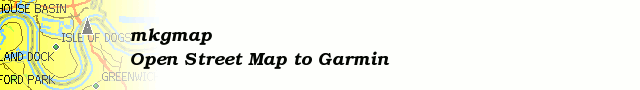# [mkgmap-dev] Overview map: tiny patch/review series

From Elrond elrond+openstreetmap.org at samba-tng.org on Tue Jun 16 19:11:02 BST 2009

```On Tue, Jun 16, 2009 at 07:58:24AM +0200, Thilo Hannemann wrote:
> Hi Elrond,
[...]
> The problem of distorted polygons does not only appear in the overview
> map, it appears at all zoom levels.
[...]

Hi,

Okay, about the DP code, I have some speculation, why it
performs bad. It's a variation on what happens for the
overview map in general.

Rounding.

Okay, let me make up an example.

Let's assume, that current resolution will round to full
units (you might think degree). And lets assume
mathematical rounding (1.4 -> 1, 1.5 -> 2)

A: (0.2, 2.4)
B: (1.2, 2.4)
C: (1.2, 2.5)

so the bare inter-vectors are:

A->B: (1.0, 0.0)
B->C: (0.0, 0.2)

Looking at that, it seems, that B is not far off from A->C.
So we drop B.

Now let's see, what the rounding makes out of the whole
story:

a = round(A): (0, 2)
b = round(B): (1, 2)
c = round(C): (1, 3)

So for not dropping B:
a->b: (1, 0)
b->c: (0, 1)

For dropping B:
a->c: (1, 1)

The distance of b to a->c is sqrt(2)/2 (0.707), so more
than unit/2.

Greetings

Elrond

```